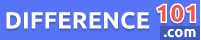Tensile Strength

If we look at tensile strength meaning: it is the maximum amount of stress which bears by an object before it breaks. Tensile strength has a specific application in the field of engineering and physics. Tensile strength is an intensive property, as it does not depend upon the size of the object which is used. The tensile strength limit comes after the elastic limit. The difference between tensile and yield strength depends upon a few factors. As yield strength is the minimum force, it causes the deformation of that object permanently. While tensile is the maximum force that an object can bear before it starts breaking. So, in this case, the force which is applied externally is greater than the forces of attraction binding that object together.WordPress database error: [Unknown column 'post_id' in 'where clause']
`SELECT * FROM wp_qss WHERE post_id = 10080`

WordPress database error: [Unknown column 'post_id' in 'where clause']
`SELECT * FROM wp_qss WHERE post_id = 10080`

WordPress database error: [Unknown column 'post_id' in 'where clause']
`SELECT * FROM wp_qss WHERE post_id = 10080`

WordPress database error: [Unknown column 'post_id' in 'where clause']
`SELECT * FROM wp_qss WHERE post_id = 10080`